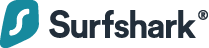# How to Split a Number into an Array in JavaScript

### Surfshark VPN: Your ultimate cyber shieldProtecting your connection is key. Surfing the web, you're exposed to many risks: data breaches, hacking attacks, & snoopers. One solution to fight them all — a VPN.

### 1. Array.from() Method

To split a number into an array in JavaScript, call the `Array.from()` method, passing the number converted to a string as the first argument, and the `Number` constructor as the second, i.e., `Array.from(String(num), Number)`. For example:

``````function splitIntoArray(num) {
return Array.from(String(num), Number);
}

const arr1 = splitIntoArray(1234);
console.log(arr1); // [ 1, 2, 3, 4 ]

const arr2 = splitIntoArray(4901);
console.log(arr2); // [ 4, 9, 0, 1 ]
``````

The static `Array` `from()` method creates a new array from an array-like object, like a `String` or a `Set`.

``````// [ '1', '2', '3', '4' ]
console.log(Array.from('1234'));
``````

The second argument we pass to `from()` is a map function that is called on every element of the array. We pass the `Number` constructor function so that each item in the array will be converted to a number.

We can do this more explicitly with the `Array` `map()` instance method:

``````const num = 1234;

// ['1', '2', '3', '4'];
const arrOfStrs = Array.from(String(num));

const arrOfNums = arrOfStrs.map((str) => Number(str));

console.log(arrOfNums); // [ 1, 2, 3, 4 ]
``````

### 2. String split() Method

We can also split a number into an array with the `String` `split()` method. To do this:

1. Convert the number to a string.
2. Call the `split()` method on the string to convert it into an array of stringified digits.
3. Call the `map()` method on this array to convert each string to a number.
``````function splitIntoArray(num) {
return String(num).split('').map(Number);
}

const arr1 = splitIntoArray(1234);
console.log(arr1); // [ 1, 2, 3, 4 ]

const arr2 = splitIntoArray(4901);
console.log(arr2); // [ 4, 9, 0, 1 ]
``````

The `String` `split()` method divides a string into an array of substrings based on the specified separator. We specify an empty string (`''`) as the separator to split the string into an array of all its characters.

``````// ['1', '2', '3', '4'];
console.log('1234'.split(''));
``````

We call `map()` on this string array, passing the `Number` constructor to convert each element to a number and create a new number array.

``````// [ 1, 2, 3, 4 ]
console.log(['1', '2', '3', '4'].map(Number));
``````

#### Tip

This:

``````// [1, 2, 3, 4]
console.log(['1', '2', '3', '4'].map(Number));``````

produces the same results as this:

``````// [ 1, 2, 3, 4 ]
console.log(['1', '2', '3', '4']
.map((str) => Number(str)));
``````

Although you might prefer to be more explicit with the second way, so you can see exactly what callback arguments you’re passing to `Number`.

## Every Crazy Thing JavaScript Does

A captivating guide to the subtle caveats and lesser-known parts of JavaScript.